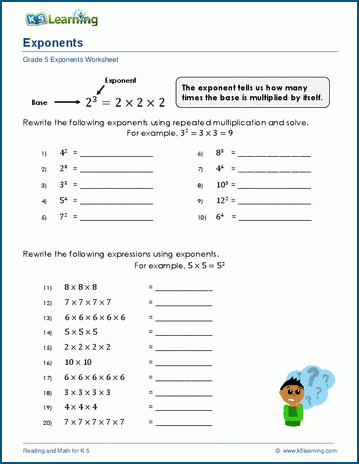# Introduction to Exponents

## Exponents as repeated multiplication

These worksheets introduce exponents as repeated multiplication of a base number.  Examples show how to expand an exponent into a multiplication expression, and how to rewrite a number being multiplied by itself as an exponent.## More exponents worksheets

Explore all of our exponents worksheets, from reading and writing simple exponents to negative exponents and equations with exponents.

## What is K5?

K5 Learning offers reading and math worksheets, workbooks and an online reading and math program for kids in kindergarten to grade 5.  We help your children build good study habits and excel in school.